# All Courses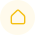DashboardAll Courses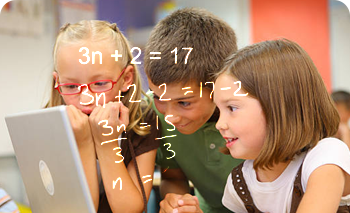\$89.99/Month

Active engagement with numbers by focusing on conceptual understanding, computational and procedural skills, and problem solving. Rational numbers, Algebraic thinking, Proportional Reasoning, Statistics, Data Analysis, Probability, and Plane and Solid Shapes.\$89.99/Month

Number and operations; Proportionality; expressions, equations, and relationships; and measurement and data. Students use concepts, algorithms, and properties of rational numbers to explore mathematical relationships and to describe increasingly complex situations\$89.99/Month

A disciplined study of Real numbers and their properties, algebraic expressions, polynomials, exponents, radicals; algebraic relations and functions, the theory of equations and inequalities with emphasis on how to solve them and graph them\$99.99/Month

### Algebra 1

Algebra foundations. Overview and history of algebra Introduction to variables Substitution and evaluating expressions. Solving equations & inequalities. Working with units. Linear equations & graphs. Forms of linear equations. Systems of equations. Inequalities (systems & graphs).Functions.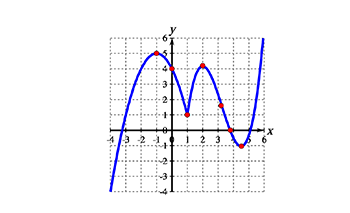\$99.99/Month

### Precalculus

Functions and Graphs. Lines and Rates of Change. Sequences and Series. Polynomial and Rational Functions. Exponential and Logarithmic Functions. Analytic Geometry. Linear Algebra and Matrices. Probability and Statistics.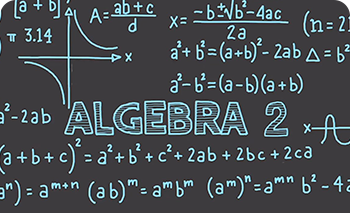\$99.99/Month

### Algebra 2

study of algebraic models, functions, graphs, linear relationships, linear relationships, matrices, linear systems, quadratic equations and functions, polynomial and polynomial functions, exponential and logarithmic functions, rational functions, quadratic relations and conic sections, sequence and series, probability and statistics, periodic functions and trigonometric identities and equations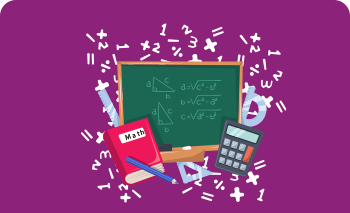\$99.99/Month

### Geometry

Angle relationships, the properties of polygons, non-polygons, polyhedral, and non-polyhedral area, volume, construction techniques, and planar transformations.\$99.99/Month

### SAT

Heart of Algebra, which focuses on the mastery of linear equations and systems.• Problem Solving and Data Analysis, which is about being quantitatively literate.• Passport to Advanced Math, which features questions that require the manipulation of complex equations.• Additional Topics in Math, including the geometry and trigonometry most relevant to college and career readiness.\$99.99/Month

### PSAT

Heart of Algebra, which focuses on the mastery of linear equations and systems.• Problem Solving and Data Analysis, which is about being quantitatively literate.• Passport to Advanced Math, which features questions that require the manipulation of complex equations.• Additional Topics in Math, including the geometry and trigonometry.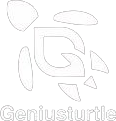Learn today achieve tomorrow!
512-222-7770info@geniusturtle.com